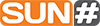# Mathematics (MAT)

### MAT 010

Math Help- 911 (2)

Small group supplemental instruction in math and study skills development. Co-requisite: *MAT 082 or *MAT 086 or *MAT 091 or *MAT 096 (MAT 122) or *MAT 097 (MAT 121). Must be taken for S/U grading. Two lecture.

### MAT 088 (4)

Pre-Algebra

Arithmetic concepts with integers, fractions, and decimals, including signed numbers.
Solving linear equations and evaluating algebraic expressions. Prerequisite: Placement. Four lecture.

### MAT 091 (4)

Beginning Algebra

Basic algebraic concepts including operations with signed numbers, exponents and radicals,
linear equations and inequalities, polynomials, and graphing. Prerequisite: MAT 088 or placement. Four lecture.

### MAT 097 (4)

Intermediate Algebra

Basic algebraic concepts, including rational expressions, functions and their graphs, radicals, quadratics, and logarithms/exponentials. Prerequisite *MAT 091 or placement. Four lecture.

### MAT 140 (5)

College Mathematics with Algebra Review

Students will examine finance, growth, probability, statistics, and common applications encountered in society. Review material will be taught just in time for when it is needed. General Education: Mathematics. Prerequisite: *MAT 091 or placement into  MAT 097 or MAT 140. Five lecture.

### MAT 142 (3)

College Mathematics

Students will examine finance, growth, probability, statistics, and common applications encountered in society. General Education: Mathematics. Prerequisite: *MAT 097  or placement.  Three lecture.

### MAT 151 (4)MAT 1151

College Algebra

College level algebra, including equations, functions, matrices, inequalities, sequences and series, and fundamental algebra theorems will be studied. Prerequisite:  MAT 097 (previously MAT 121) or placement.General Education: Mathematics. Four lecture. Fall.

### MAT 160 (3)MAT 1160

Introduction to Statistics

Introduction to Statistics involves statistical tools and techniques used in applications. Includes collection of data, graphical and descriptive statistics, probability and probability distributions, point and interval estimates of population parameters, hypothesis testing, and correlation and regression. Prerequisite: *Any college level math or placement. General Education: Mathematics. Three lecture. Fall, Spring

### MAT 172 (3)

Finite Mathematics

Various analytical methods used in business and social sciences, including algebra review, functions and modeling, systems of linear equations, matrices, linear programming, mathematics of finance, probability, and combinations. Prerequisite: *MAT 151 or placement. Three lecture. Fall, Spring.

### MAT 187 (5)MAT 1187

Pre-Calculus

College level algebra and trigonometric topics to prepare for calculus. Functions, equations, and inequalities, trigonometry, and fundamental algebra theorems will be studied. General Education: Mathematics. Prerequisite: *MAT 097 or placement. Five lecture.

### MAT 211 (4)MAT 2212

Integral and differential calculus, including multidimensional, with business and social science applications. General Education: Mathematics. Prerequisite: MAT 151 or placement. General Education: Mathematics. Four lecture. Spring.

### MAT 220 (5)MAT 2220

Calculus & Analytic Geometry I

Limits, continuity, differential, and integral operations on algebraic and trigonometric functions and applications. Prerequisite: *MAT 187 or placement. General Education: Mathematics. Five lecture.

### MAT 230 (5)MAT 2230

Calculus & Analytic Geometry II

Applications and methods of integration, Taylor polynomials and series, differential equations, multi-variable functions and vectors. Prerequisite: *MAT 220. General Education: Mathematics. Five lecture.

### MAT 241 (4)MAT 2241

Calculus & Analytic Geometry III

Multidimensional calculus. Includes conic sections, polar coordinates, partial derivatives, gradients, directional derivatives, extrema, multiple and iterated integrals, vector calculus, line integrals, and Green’s Theorem. Prerequisite: *MAT 230. General Education: Mathematics. Four lecture.

### MAT 261 (4)MAT 2262

Differential Equations

Introduction to ordinary differential equations. Includes first order equations, higher order linear equations, applications of first and second order equations, series solutions, Laplace transforms, and systems of linear differential equations. General Education: Mathematics. Prerequisite: *MAT 230. Four lecture.

### MAT 198/298 (1–6)

Special Topics

Designed to meet the needs of an individual(s) who has an interest in pursuing an original topic in an instructional area under faculty supervision. One to six variable credit hours.

*Course has additional pre or co requisite(s)